Question

# A solution of which compound will be in the buret during your experiment? NaOH HCl HOAc...

1. A solution of which compound will be in the buret during your experiment?

1. NaOH
2. HCl
3. HOAc
4. EtOAc
5. EtOH
1. You will use titration to determine the equilibrium concentration of one component of this chemical reaction. Which component will you be determining the concentration of?
6. HOAc (acetic acid)
7. H2O     (water)
8. EtOAc (ethyl acetate)
9. EtOH   (ethyl alcohol)
10. HCl (hydrochloric acid)
1. Recall how titration works. If 17.51mL of 0.0985M NaOH is need to achieve the endpoint of a titration with KHP (potassium hydrogen phthalate), how many moles of KHP were originally in the sample?

NaOH (a base) and KHP (an acid) react in a 1:1 ratio.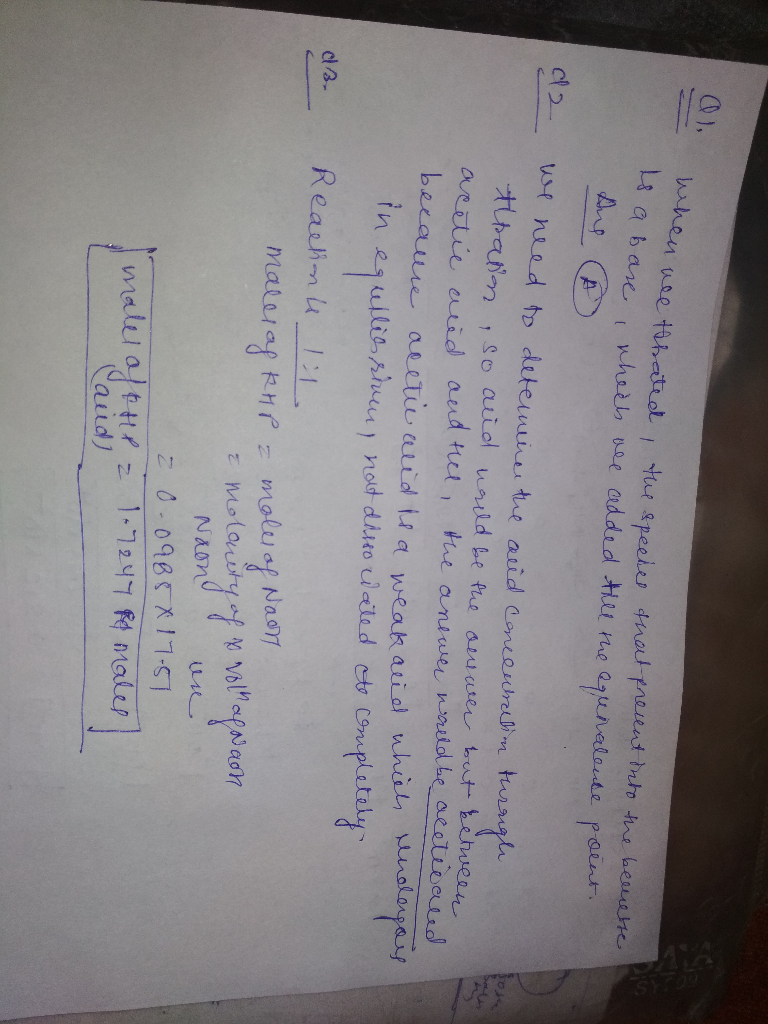#### Earn Coins

Coins can be redeemed for fabulous gifts.

Similar Homework Help Questions
• ### 1. A solution of sodium hydroxide (NaOH) was standardized against potassium hydrogen phthalate (KHP). A known...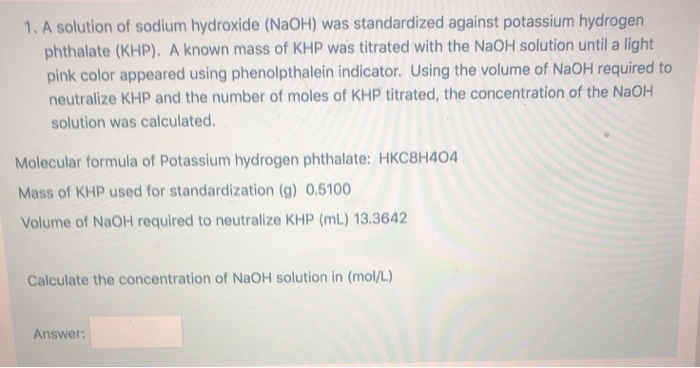1. A solution of sodium hydroxide (NaOH) was standardized against potassium hydrogen phthalate (KHP). A known mass of KHP was titrated with the NaOH solution until a light pink color appeared using phenolpthalein indicator. Using the volume of NaOH required to neutralize KHP and the number of moles of KHP titrated, the concentration of the NaOH solution was calculated. Molecular formula of Potassium hydrogen phthalate: HKC8H404 Mass of KHP used for standardization (g) 0.5100 Volume of NaOH required to neutralize...

• ### Name Section/CRN EXPERIMENT 9 POTENTIOMETRIC DETERMINATION OF AN EQUILIBRIUM CONSTANT PRE-LABORATORY QUESTIONS . The following preparatory...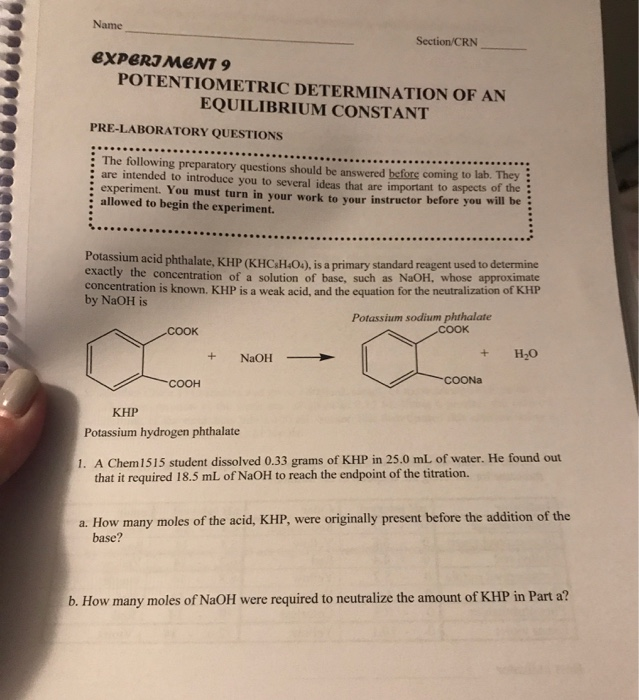Name Section/CRN EXPERIMENT 9 POTENTIOMETRIC DETERMINATION OF AN EQUILIBRIUM CONSTANT PRE-LABORATORY QUESTIONS . The following preparatory questions should be answered before coming to b. are intended to introduce you to several ideas that are important to aspects of the experiment. You must turn in your work to your instructor before you allowed to begin the experiment. Potassium acid phthalate, KHP (KHC.H.O.), is a primary standard reagent used to determine exactly the concentration of a solution of base, such as NaOH,...

• ### Data Standard solution: NaOH concentration 0.25 M Volume of HCl used (Va) Initial NaOH buret reading...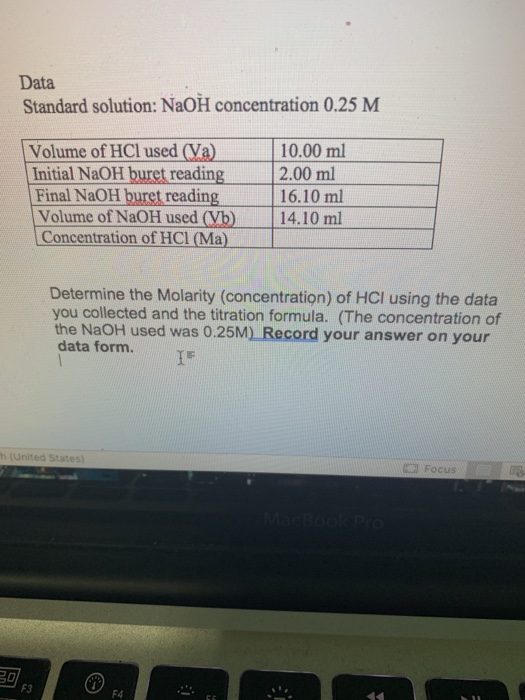Data Standard solution: NaOH concentration 0.25 M Volume of HCl used (Va) Initial NaOH buret reading Final NaOH buret reading Volume of NaOH used (Vb) Concentration of HCl (Ma) 10.00 ml 2.00 ml 16.10 ml 14.10 ml Determine the Molarity (concentration) of HCI using the data you collected and the titration formula. (The concentration of the NaOH used was 0.25M) Record your answer on your data form. United States Focus 17. It takes 75ml of a 2.5M HCl solution to...

• ### Standardization of NaOH: Acid Base Titration Objective: In this lab, you will accurately determine the concentration...

Standardization of NaOH: Acid Base Titration Objective: In this lab, you will accurately determine the concentration of a solution of sodium hydroxide (NaOH) using a 0.500M potassium hydrogen phthalate (KHP) standard solution. Background: Acid–Base Titrations When an acid reacts with a base, a neutralization reaction occurs. The H+ ions from the acid and the HO– ions from the base combine to form water and are therefore neutralized. The other product of reaction is a salt. For example, hydrochloric acid reacts...

• ### CHEM LAB hello can someone help me come up with a procedure for this lab it...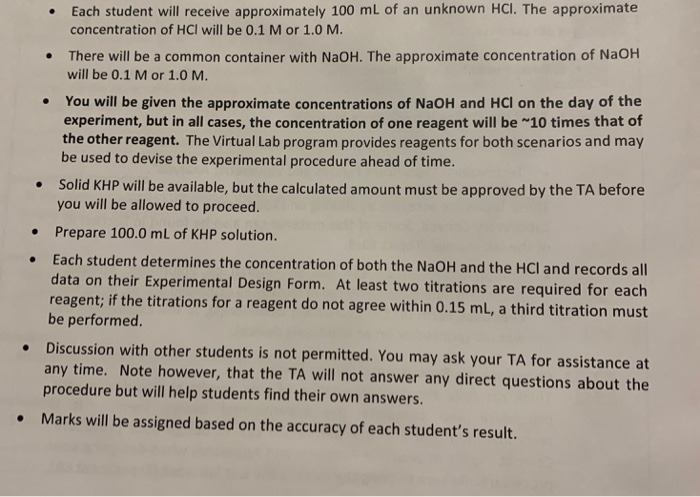CHEM LAB hello can someone help me come up with a procedure for this lab it is an standarization of acid and base solution • Each student will receive approximately 100 mL of an unknown HCl. The approximate concentration of HCl will be 0.1 M or 1.0 M. There will be a common container with NaOH. The approximate concentration of NaOH will be 0.1 M or 1.0 M. You will be given the approximate concentrations of NaOH and HCl on...

• ### STR Data Standard solution: NaOH concentration 025M volume of initial NaOH buret reading final NaOhH buret readingG TUTHL volume of NaOH used Concentration of HCI Calculations(Show all work and r...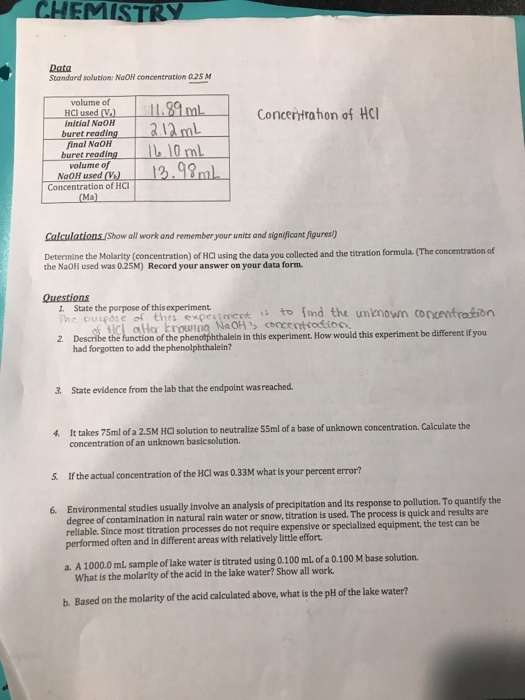STR Data Standard solution: NaOH concentration 025M volume of initial NaOH buret reading final NaOhH buret readingG TUTHL volume of NaOH used Concentration of HCI Calculations(Show all work and remember your units and significant figures) Determine the Molarity (concentration) of HCl using the data you collected and the titration formula. (The concentration of the NaOH used was 0.25M) Record your answer on your data form. Questions 1. State the purpose of this experiment The ouredse of thrs expetmeot bn of...

• ### Name Section/CRN EXPERIMENT 9 POTENTIOMETRIC DETERMINATION OF AN EQUILIBRIUM CONSTANT PRE-LABORATORY QUESTIONS The following preparatory questions...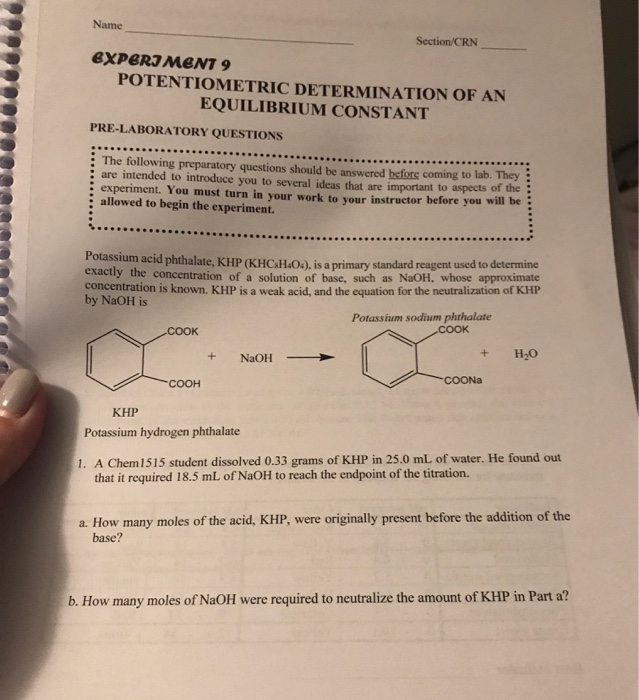Name Section/CRN EXPERIMENT 9 POTENTIOMETRIC DETERMINATION OF AN EQUILIBRIUM CONSTANT PRE-LABORATORY QUESTIONS The following preparatory questions should be answered before coming to lab. They are intended to introduce you to several jdeas that are important to aspects of the experiment. You must turn in your work to your instructor before you will be allowed to begin the experiment. Potassium acid phthalate, KHP (KHC4H&O4), is a primary standard reagent used to determine exactly the concentration of a solution of base, such...

• ### Materials: NaOH MW = 40 g/mL, KHP - potassium hydrogen phthalate, KHC8H404, MW = 204.23 g/mol....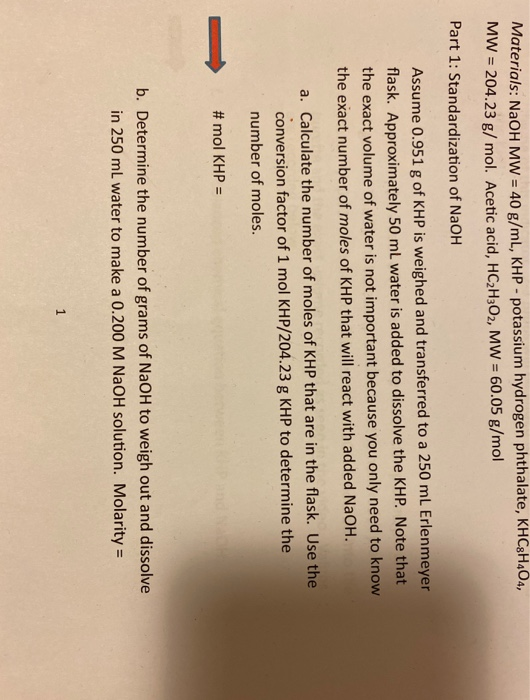Materials: NaOH MW = 40 g/mL, KHP - potassium hydrogen phthalate, KHC8H404, MW = 204.23 g/mol. Acetic acid, HC2H302, MW = 60.05 g/mol Part 1: Standardization of NaOH Assume 0.951 g of KHP is weighed and transferred to a 250 mL Erlenmeyer flask. Approximately 50 ml water is added to dissolve the KHP. Note that the exact volume of water is not important because you only need to know the exact number of moles of KHP that will react with...

• ### Materials: NaOH MW = 40 g/mL, KHP - potassium hydrogen phthalate, KHC8H404, MW = 204.23 g/mol....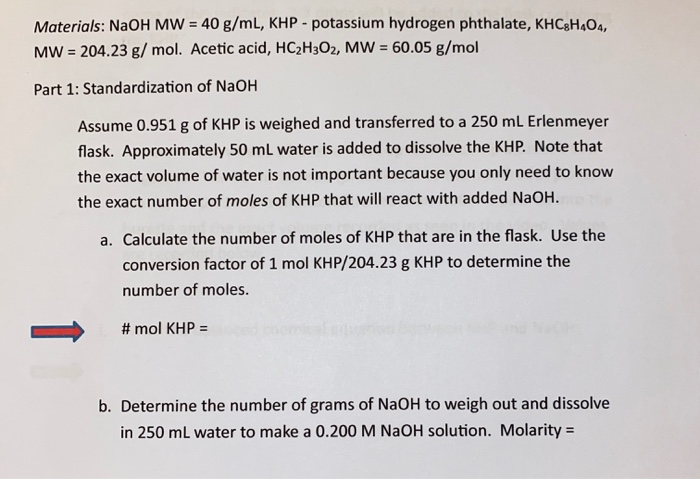Materials: NaOH MW = 40 g/mL, KHP - potassium hydrogen phthalate, KHC8H404, MW = 204.23 g/mol. Acetic acid, HC2H302, MW = 60.05 g/mol Part 1: Standardization of NaOH Assume 0.951 g of KHP is weighed and transferred to a 250 mL Erlenmeyer flask. Approximately 50 ml water is added to dissolve the KHP. Note that the exact volume of water is not important because you only need to know the exact number of moles of KHP that will react with...

• ### Section DATA SHEET: EXPERIMENT 16 Date A. Determination of NaOH Concentration 1. Molarity of HCl solution...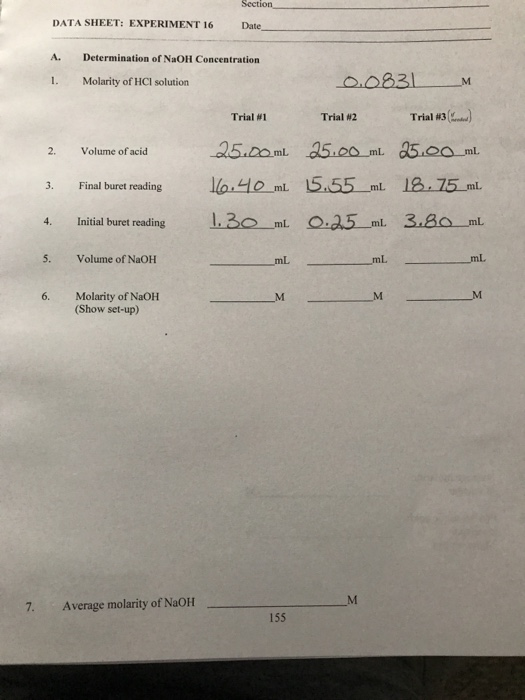Section DATA SHEET: EXPERIMENT 16 Date A. Determination of NaOH Concentration 1. Molarity of HCl solution M Trial #1 Volume of acid 0.0831 Trial #2 Trial #3(...) 25.00 ml 25.00_ml 25.00 ml 160.40 ml 5.55_ml 18.75 ml 1.30_mi 0.25_ml. 3.80 ml 3. Final buret reading Initial buret reading 5. Volume of NaOH - 6. Molarity of NaOH (Show set-up) 7. Average molarity of NaOH Analysis of Vinegar (Acetic Acid) Advertised % Acetic Acid Trial #1 Trial #2 Trial #3(...) Volume...